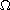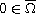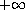Electron. J. Diff. Equ., Vol. 2015 (2015), No. 76, pp. 1-28.

### Mixed interior and boundary peak solutions of the Neumann problem for the Henon equation inYibin Zhang, Haitao Yang

Abstract:
Letbe a bounded domain inwith smooth boundary and, we study the Neumann problem for the Henon equationwheredenotes the outer unit normal vector to,and p is a large exponent. In a constructive way, we show that, as p approaches, such a problem has a family of positive solutions with arbitrarily many interior and boundary spikes involving the origin. The same techniques lead also to a more general result on Henon-type weights.

Submitted October 14, 2014. Published March 26, 2015.
Math Subject Classifications: 35J25, 35J20, 58K05.
Key Words: Mixed interior and boundary peak solutions; Henon-type weight; large exponent; Lyapunov-Schmidt reduction process.

Show me the PDF file (404 KB), TEX file, and other files for this article.Yibin Zhang College of Sciences, Nanjing Agricultural University Nanjing 210095, China email: yibin10201029@njau.edu.cn Haitao Yang Department of Mathematics, Zhejiang University Hangzhou 310027, China email: htyang@css.zju.edu.cn